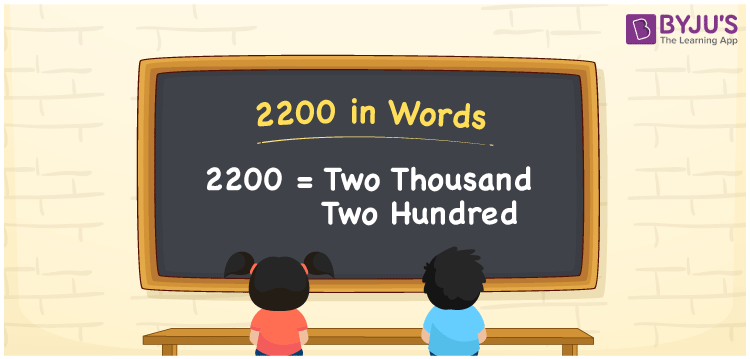# 2200 in Words

2200 in words is written as “Two thousand two hundred”. In Maths, 2200 is a cardinal number that expresses a quantity. To write a cheque for Rs.2200, we can write in words as Rupees Two thousand two hundred. Learn more about Numbers In Words and writing the number names in English at BYJU’S.

 2200 in Words Two thousand two hundred Two thousand two hundred in Numbers 2200

## 2200 in English Words

To make it easy for children to learn the names of the numbers we have represented the chart below. It shows the name of 2200 in English words.## How to Write 2200 in Words?

The number 2200 in words can be written using a place value system, where we can identify the position of each digit of the original number. Since 2200 is a four-digit number, thus,

 Thousands Hundreds Tens Ones 2 2 0 0

From the above table,

2 → Thousands

2 → Hundreds

0 → Tens

0 → Ones

Hence, when we read the number from right to left, it is given by Two thousand two hundred.

### Expanded Form of 2200

We can write the expanded form as:

2 x Thousand + 2 × Hundred + 0 × Ten + 0 × One

= 2 x 1000 + 2 × 100 + 0 × 10 + 0 × 1

= 2000 + 200 + 0 + 0

= Two thousand two hundred

2200 is a whole number that is succeeded by 2199 and preceded by 2201. Learn more about the number 2200 below:

• 2200 in Words – Two thousand two hundred
• Is 2200 an odd number? – No
• Is 2200 an even number? – Yes
• Is 2200 a perfect square number? – No
• Is 2200 a perfect cube number? – No
• Is 2200 a prime number? – No
• Is 2200 a composite number? – Yes

## Frequently Asked Questions on 2200 in words

Q1

### What is 2200 in words?

2200 in words is given by Two thousand two hundred.
Q2

### What is the rule to write 2200 in words?

To write the 2200 in words, we should use place value rule, where the position of each digit helps in determining the name of the number.
Q3

### What is the value of 2200 + 100 in words?

2200 + 100 = 2300, i.e., Two thousand three hundred in words.